Mixed spikes of uranium and plutonium have been prepared for the determination of uranium and plutonium in dissolved MOX solution by isotope dilution mass spectrometry. Enriched uranium metal NBL CRM116 and plutonium metal NBL CRM126 were accurately weighed and then dissolved in nitric acid, respectively. Their dissolved solutions were mixed in a mass ratio of 1 to 2. The preparation values of uranium and plutonium were 1.0530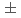0.0008 mg/g (k=2) of uranium with a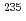U relative mass fraction of 93.114 wt% and 2.00460.0019 mg/g (k=2) of plutonium with a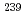Pu relative mass fraction of 97.934 wt%, respectively. The concentrations of uranium and plutonium in spike were confirmed by reverse isotope dilution mass spectrometry using tracer of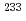U and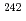Pu. Finally, the prepared spike was validated by parallel analysis of simulated sample of dissolved MOX solution. This spike was applied to measure the uranium and plutonium amount content of dissolved MOX solutions using isotope dilution mass spectrometry.